Case Based Questions (MCQ)

Chapter 13 Class 12 Probability
Serial order wise

## From this situation answer the following: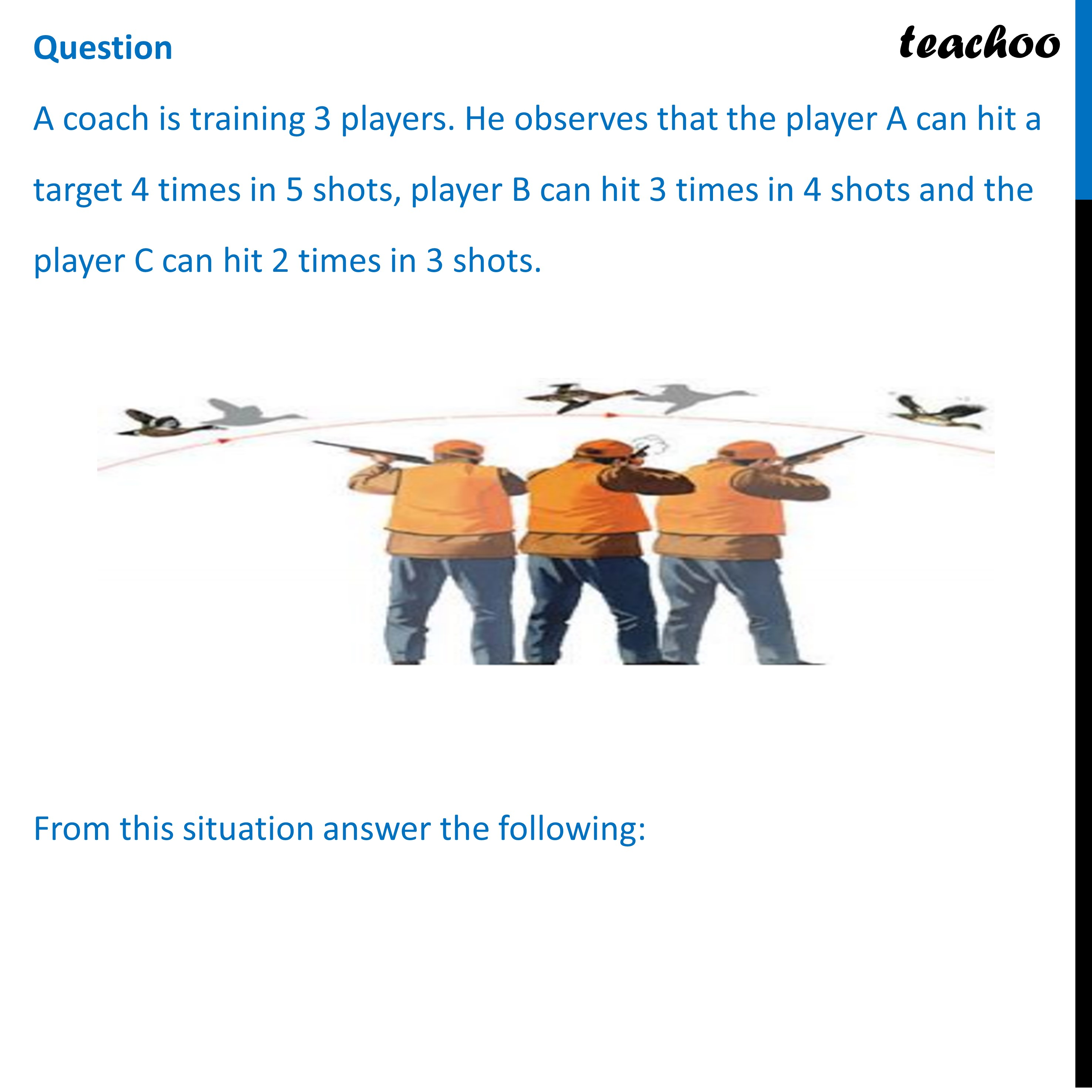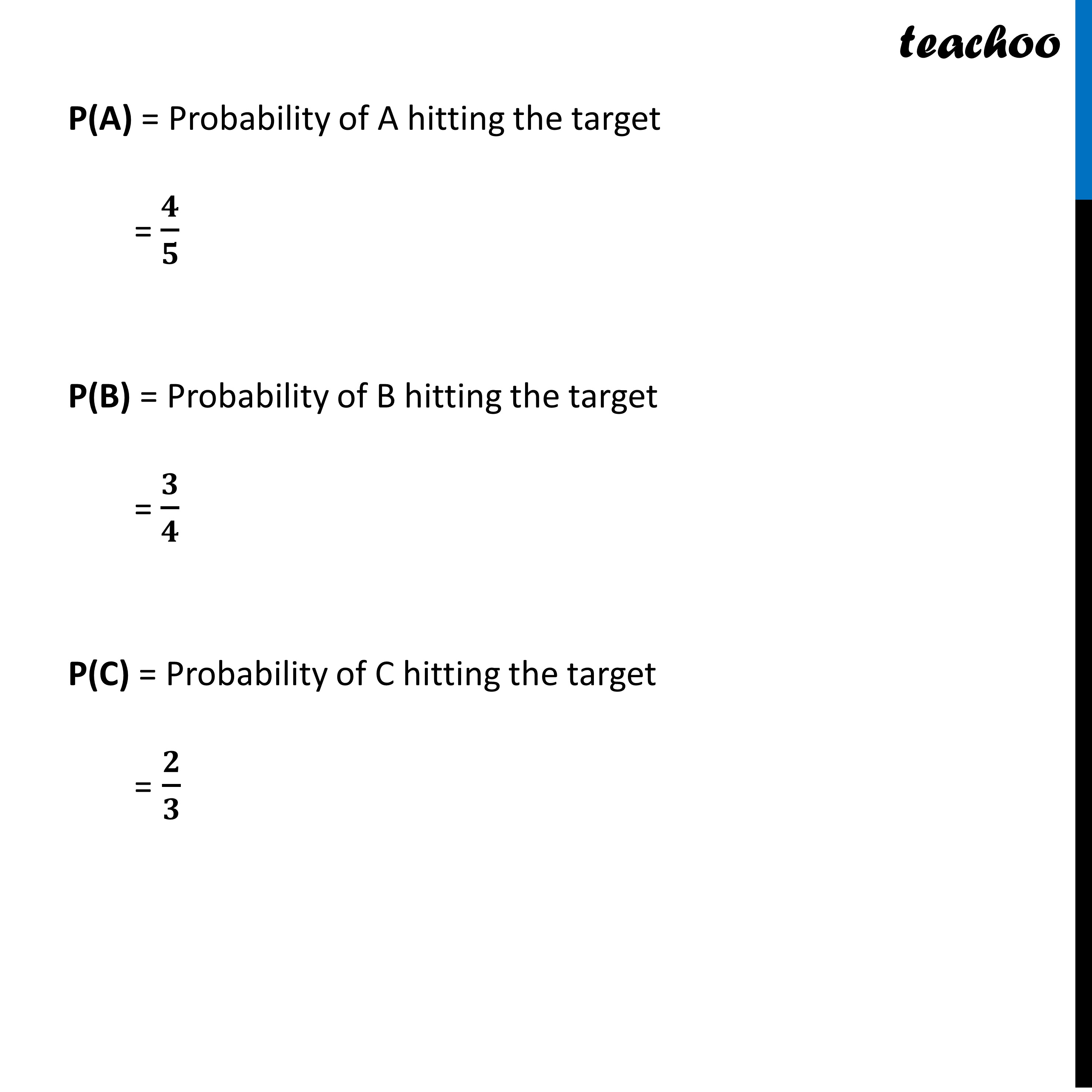## (d) 1/5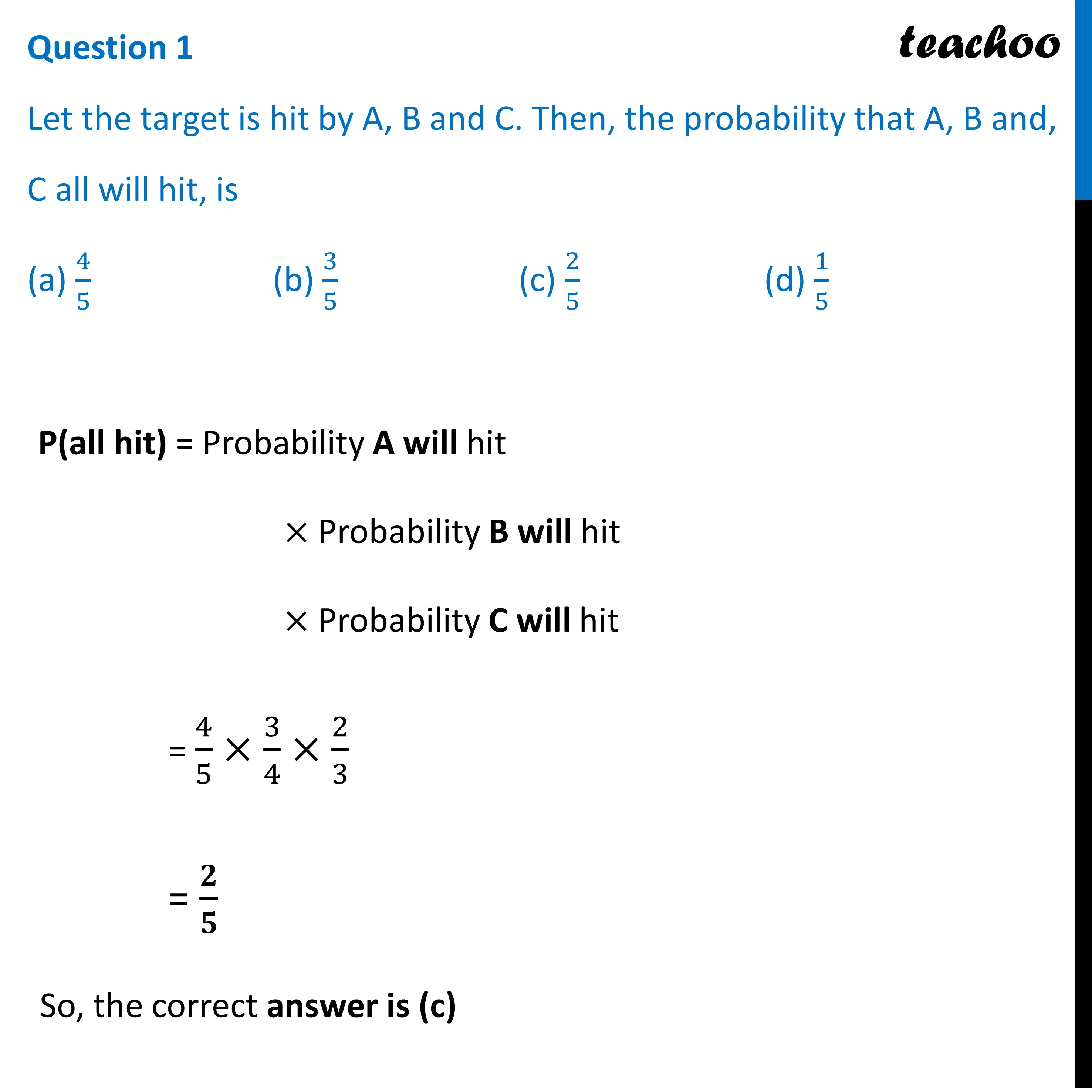## (d) 4/10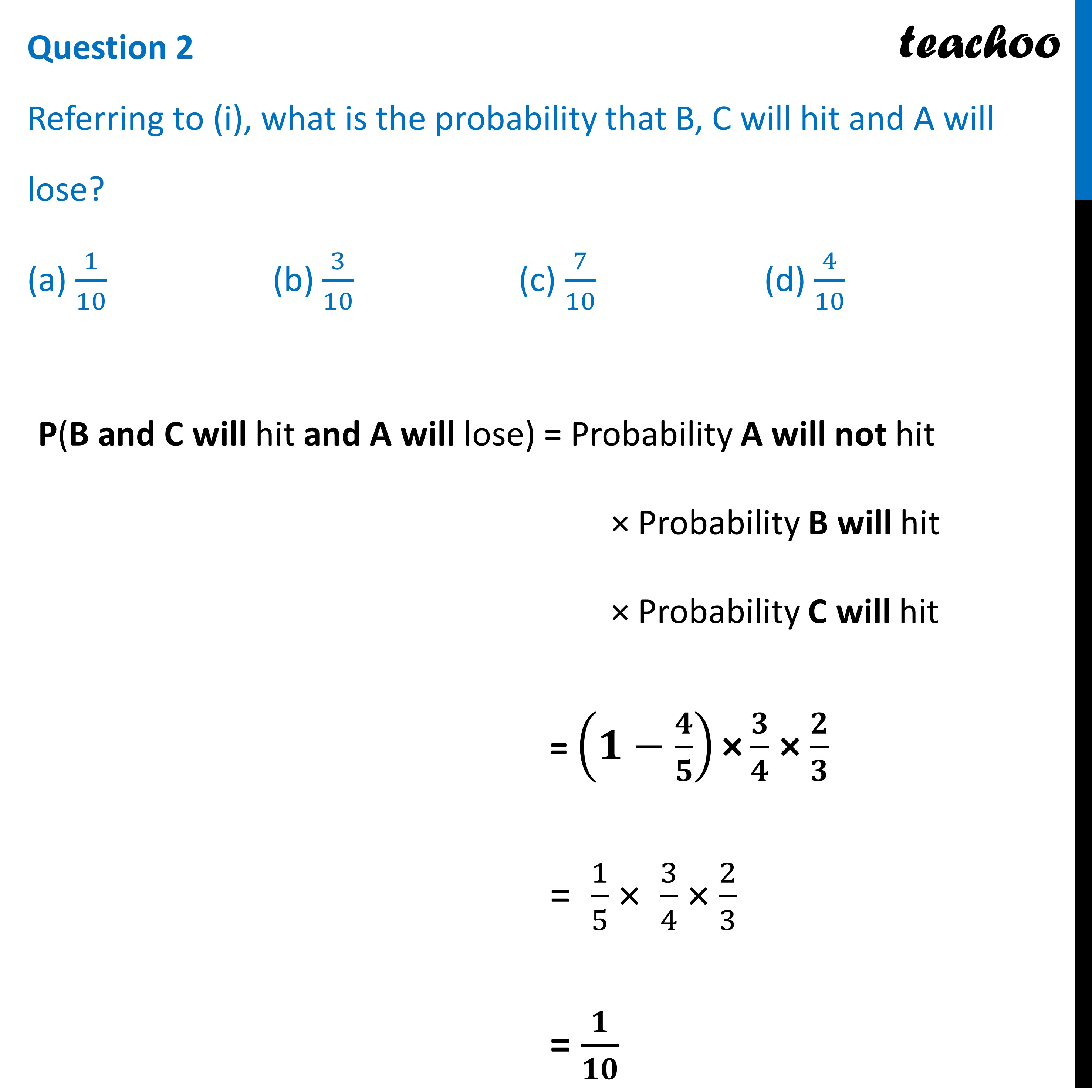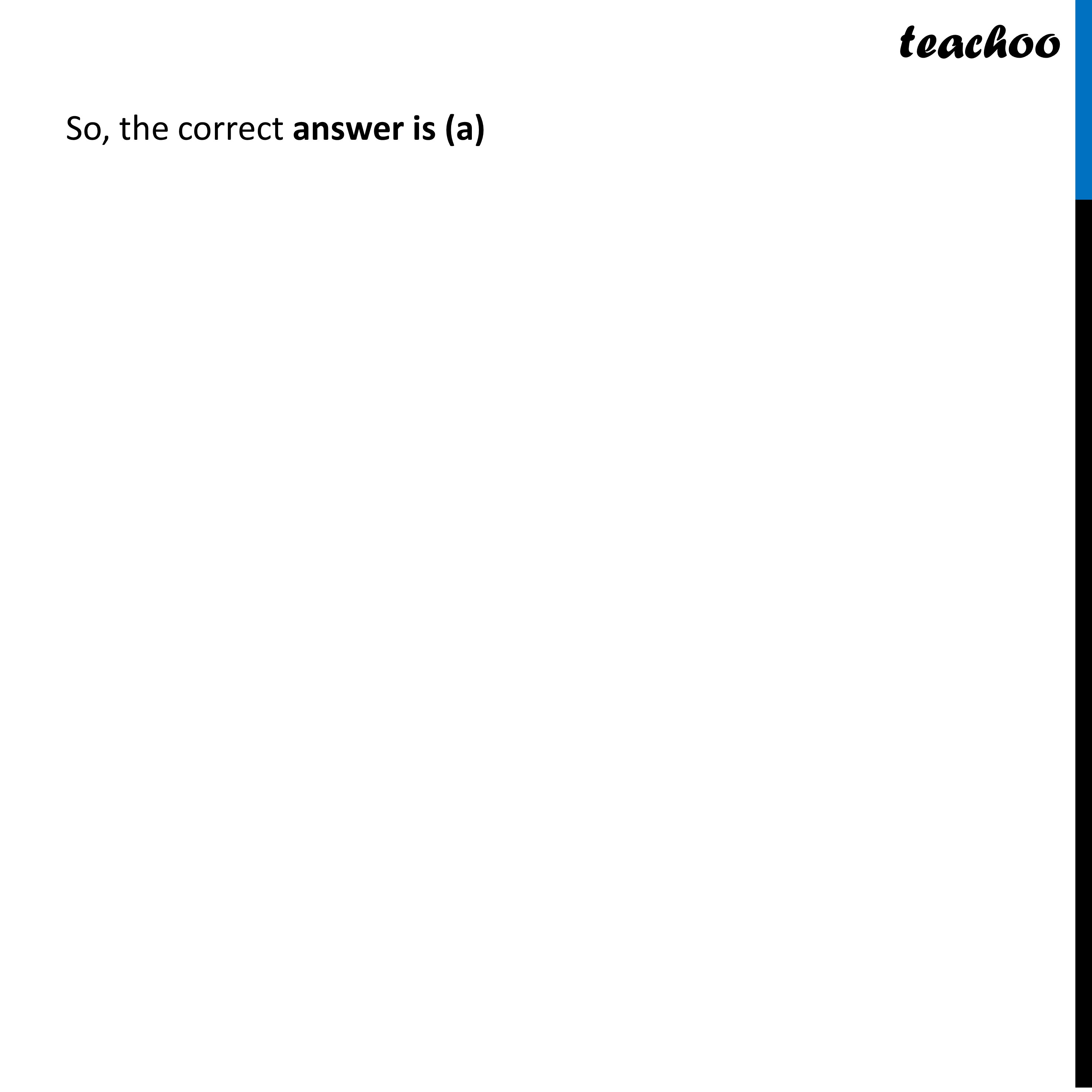## (d) 13/30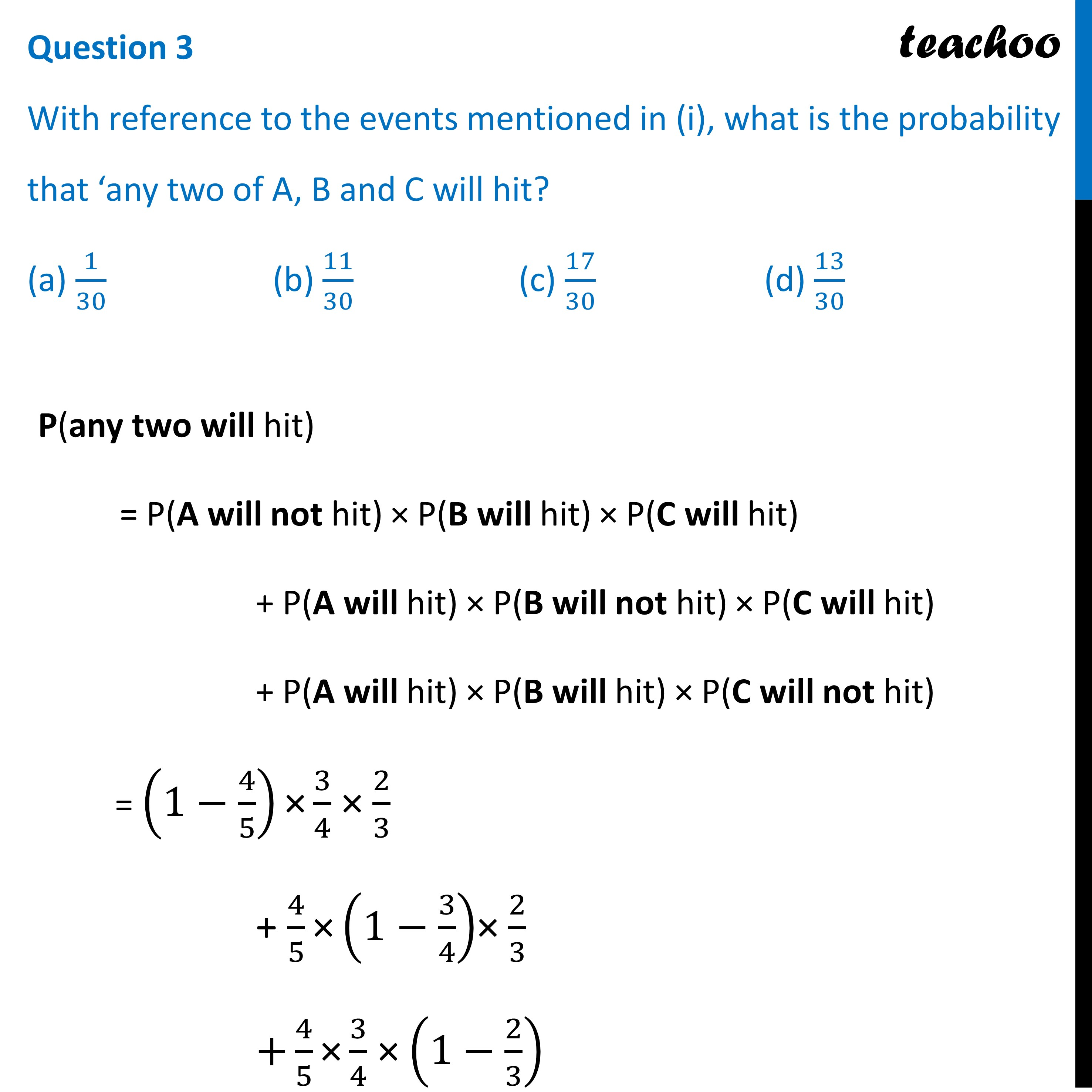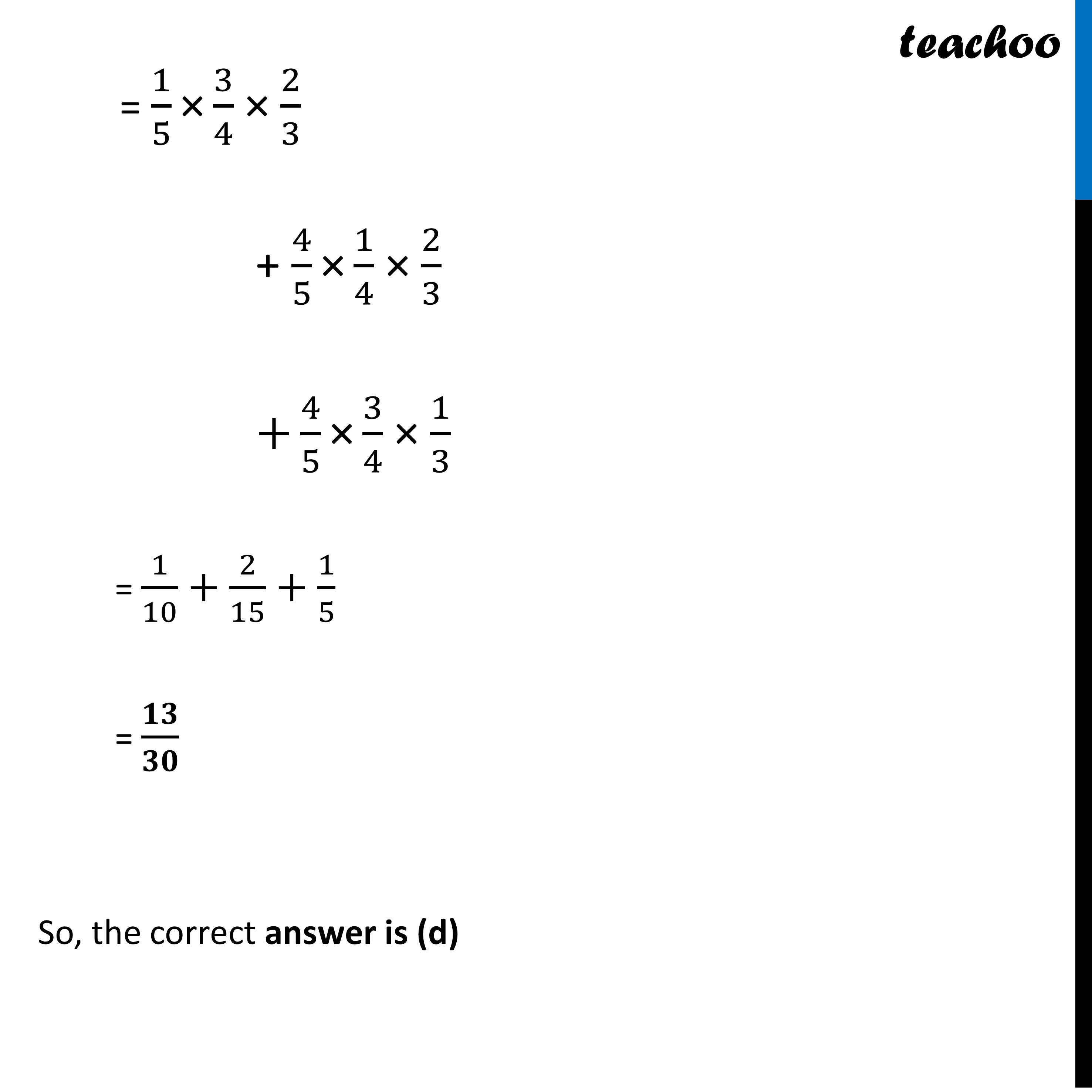## (d) 2/15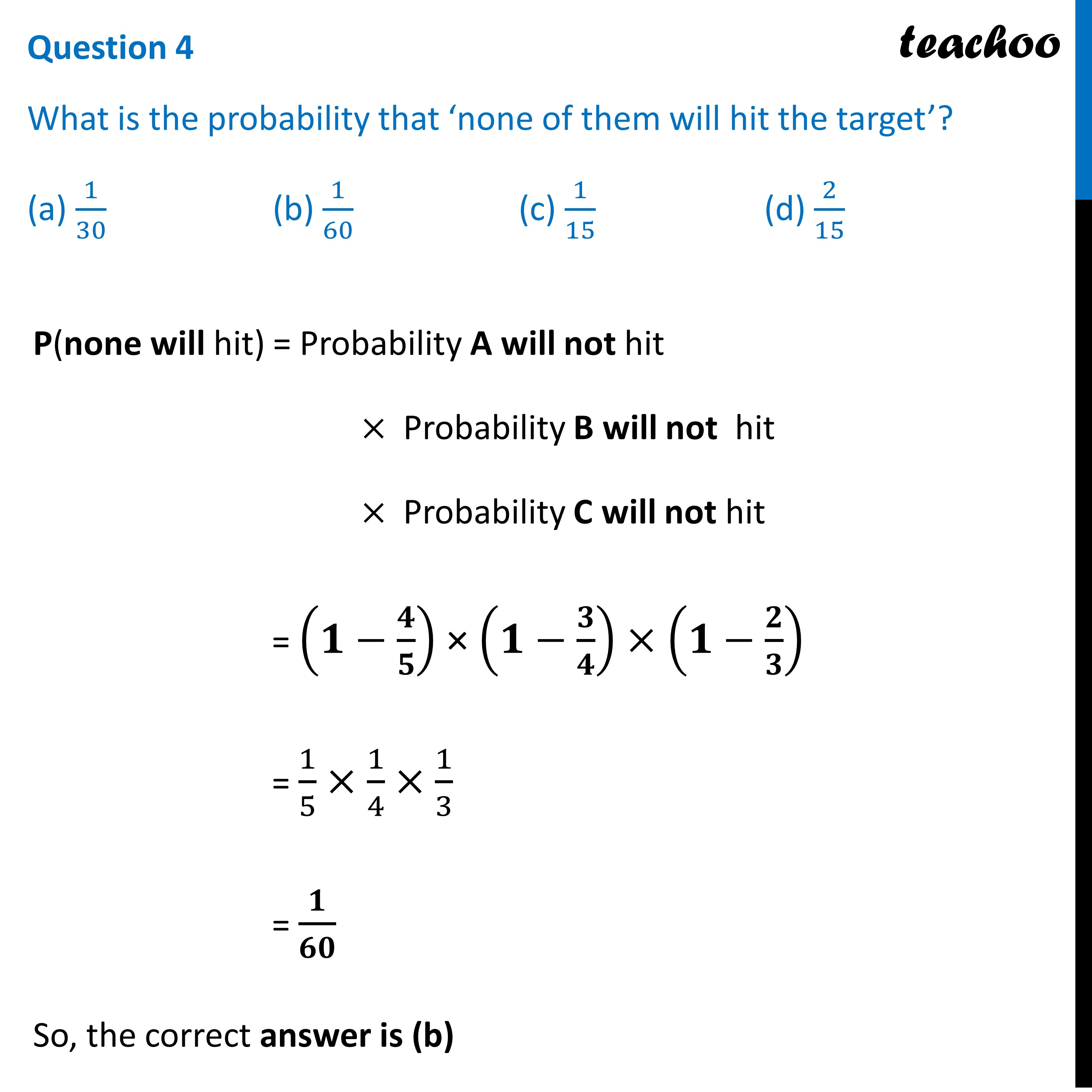##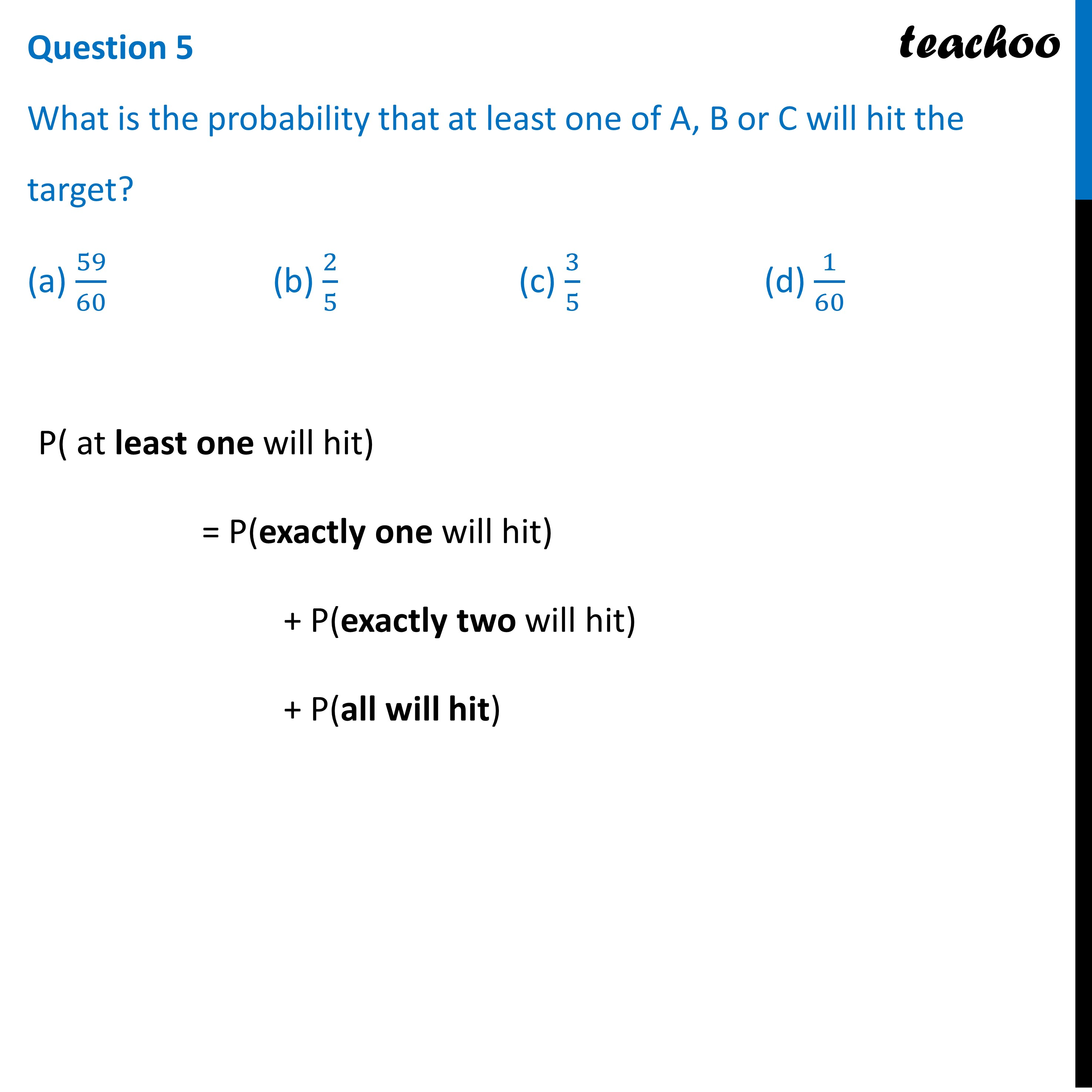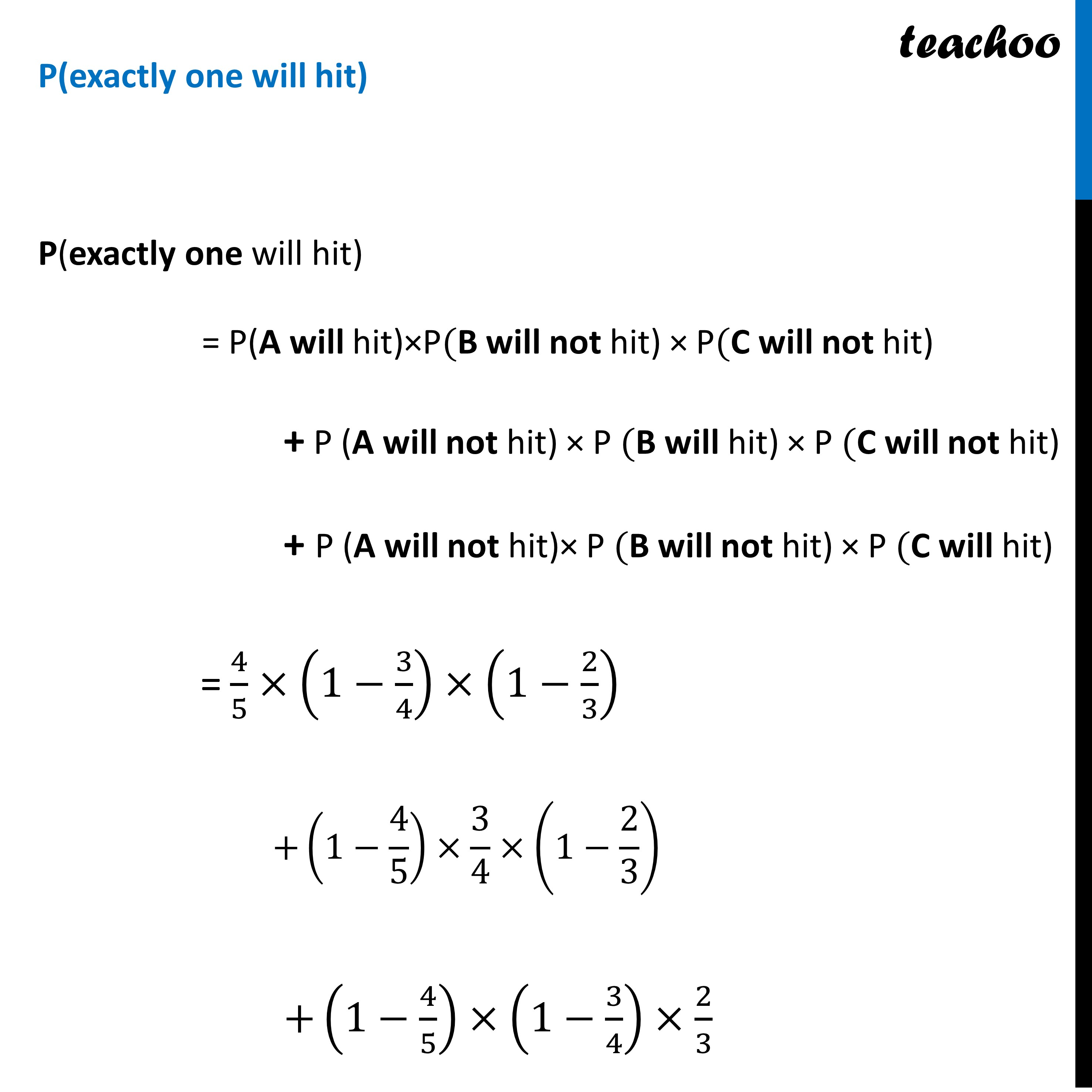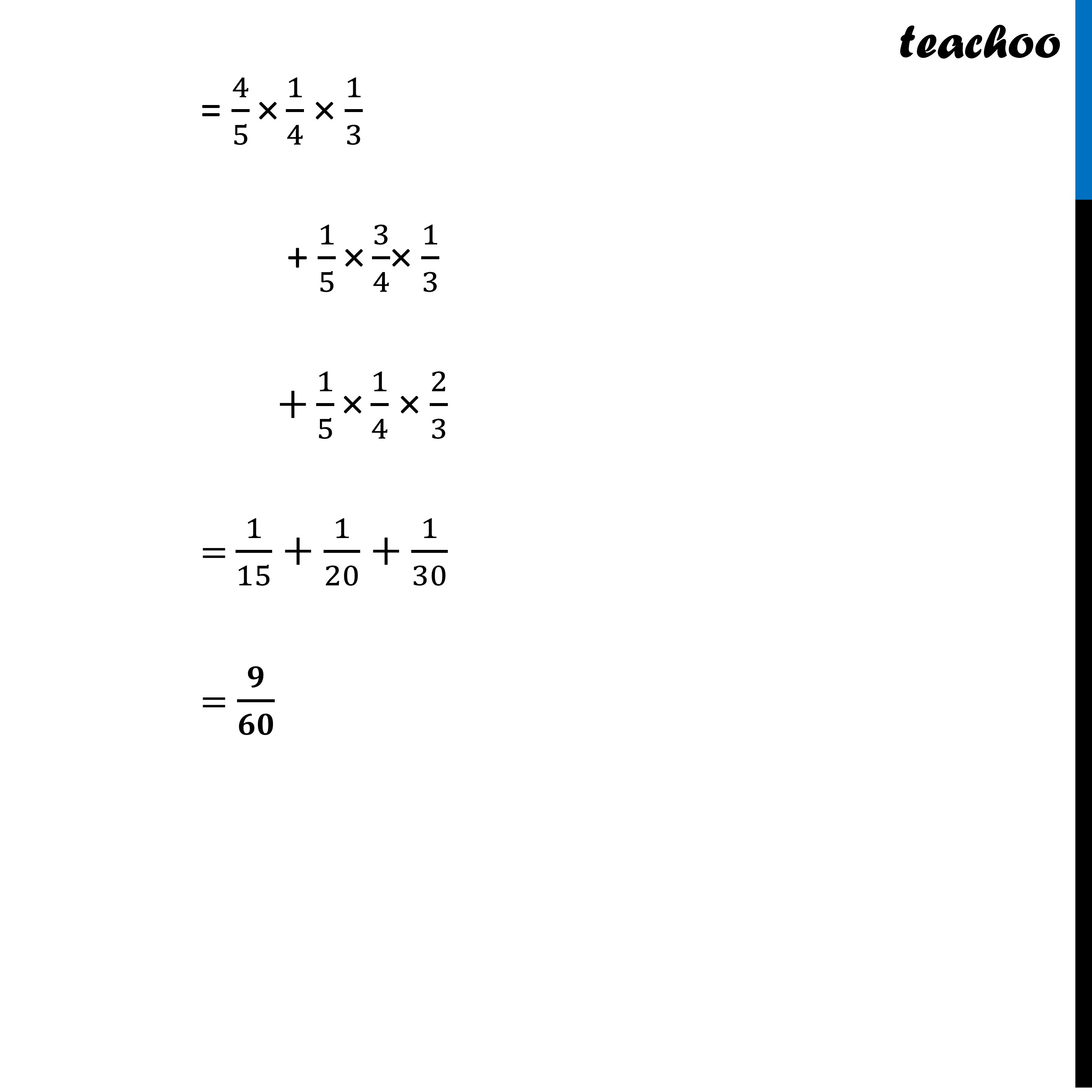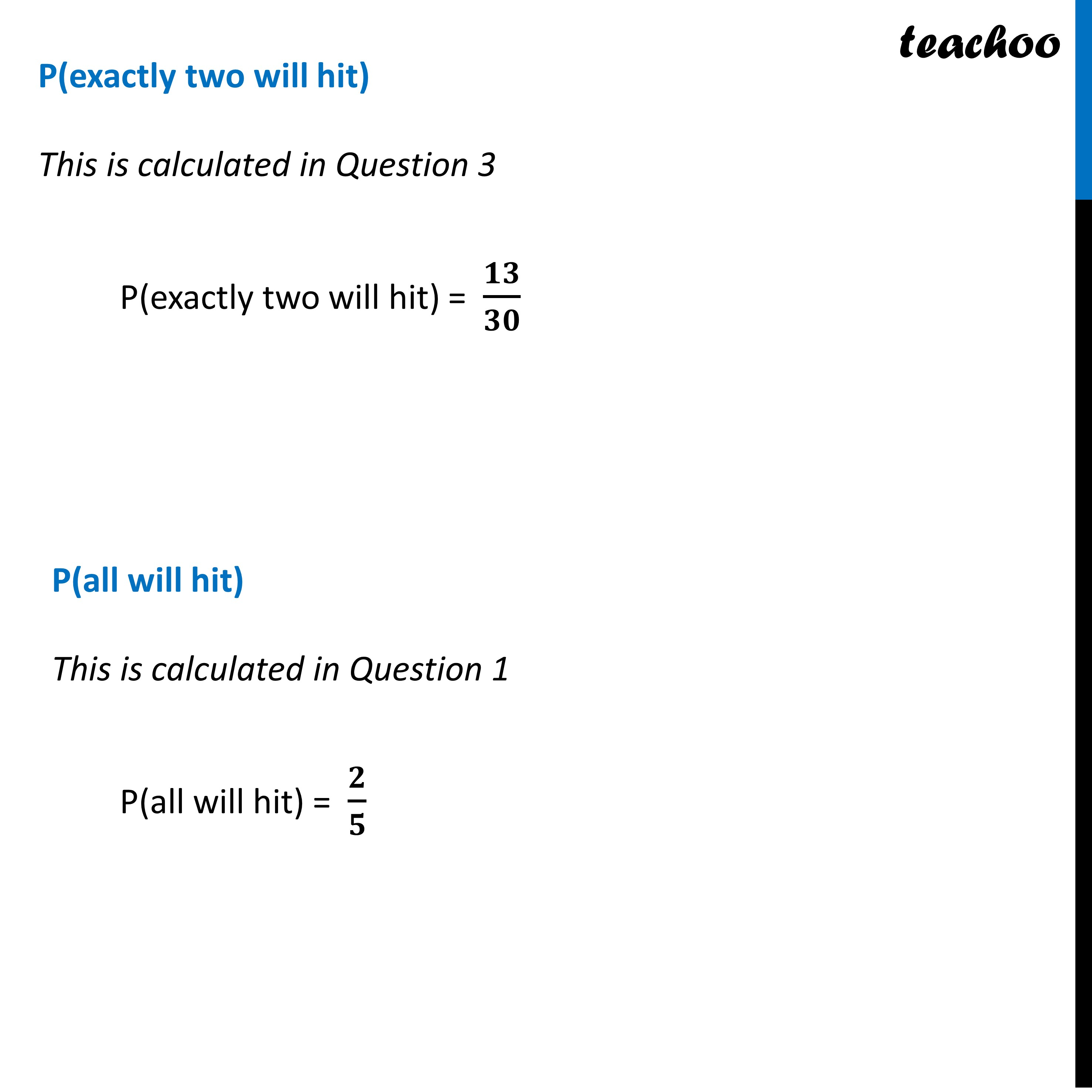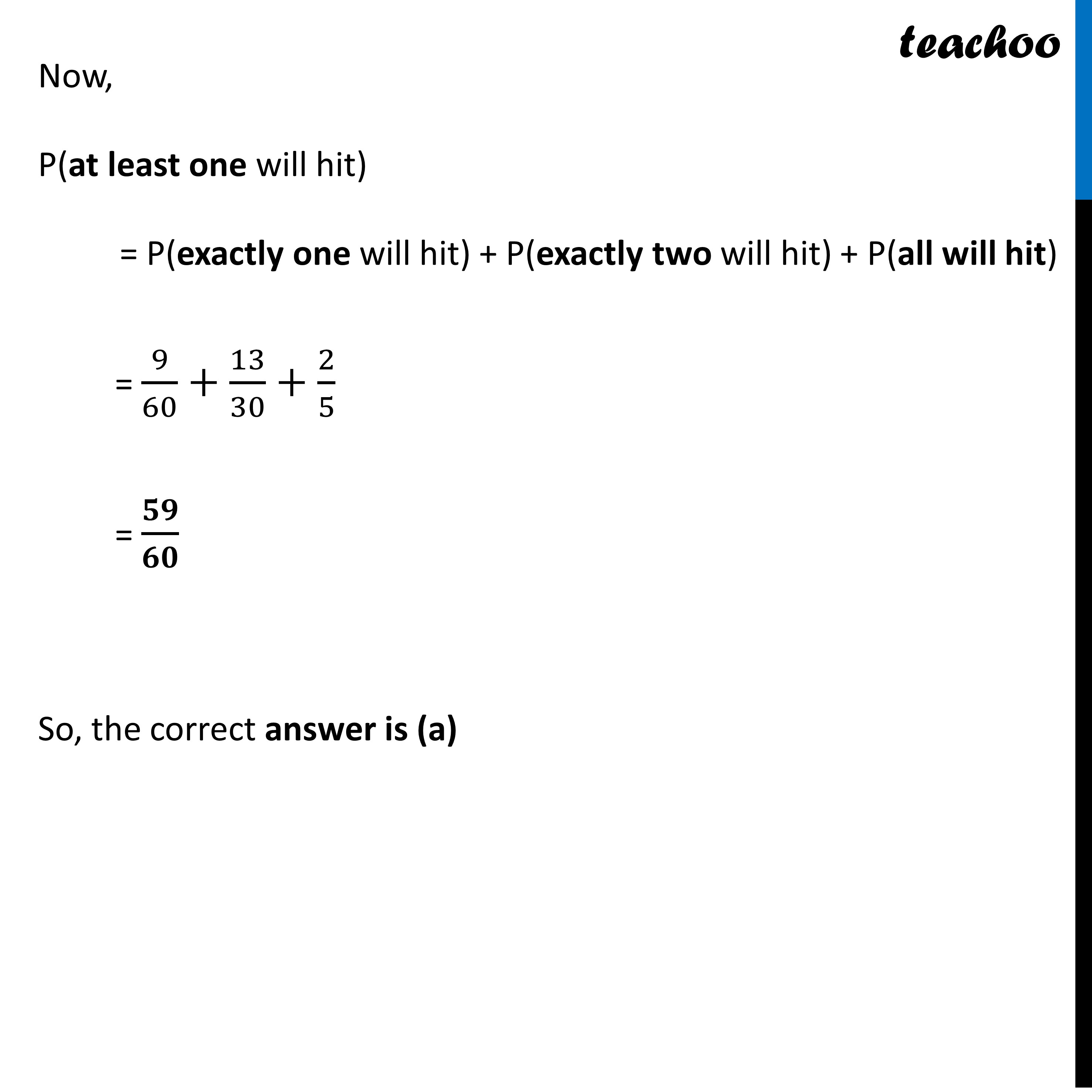Get live Maths 1-on-1 Classs - Class 6 to 12

### Transcript

Question A coach is training 3 players. He observes that the player A can hit a target 4 times in 5 shots, player B can hit 3 times in 4 shots and the player C can hit 2 times in 3 shots. From this situation answer the following:P(A) = Probability of A hitting the target = 𝟒/𝟓 P(B) = Probability of B hitting the target = 𝟑/𝟒 P(C) = Probability of C hitting the target = 𝟐/𝟑 Question 1 Let the target is hit by A, B and C. Then, the probability that A, B and, C all will hit, is (a) 4/5 (b) 3/5 (c) 2/5 (d) 1/5P(all hit) = Probability A will hit × Probability B will hit × Probability C will hit = 4/5 × 3/4 × 2/3 = 𝟐/𝟓 So, the correct answer is (c) Question 2 Referring to (i), what is the probability that B, C will hit and A will lose? (a) 1/10 (b) 3/10 (c) 7/10 (d) 4/10P(B and C will hit and A will lose) = Probability A will not hit × Probability B will hit × Probability C will hit = (𝟏−𝟒/𝟓)"×" 𝟑/𝟒 × 𝟐/𝟑 = 1/5 × 3/4 × 2/3 = 𝟏/𝟏𝟎 So, the correct answer is (a) Question 3 With reference to the events mentioned in (i), what is the probability that ‘any two of A, B and C will hit? (a) 1/30 (b) 11/30 (c) 17/30 (d) 13/30 P(any two will hit) = P(A will not hit) × P(B will hit) × P(C will hit) + P(A will hit) × P(B will not hit) × P(C will hit) + P(A will hit) × P(B will hit) × P(C will not hit) = (1−4/5)"×" 3/4 × 2/3 + 4/5 "×" (1−3/4)× 2/3 +4/5 "×" 3/4 × (1−2/3) = 1/5 "×" 3/4 × 2/3 + 4/5 "×" 1/4 × 2/3 +4/5 "×" 3/4 × 1/3 = 1/10+2/15+1/5 = 𝟏𝟑/𝟑𝟎 So, the correct answer is (d) Question 4 What is the probability that ‘none of them will hit the target’? (a) 1/30 (b) 1/60 (c) 1/15 (d) 2/15P(none will hit) = Probability A will not hit × Probability B will not hit × Probability C will not hit = (𝟏−𝟒/𝟓) × (𝟏−𝟑/𝟒)×(𝟏−𝟐/𝟑) = 1/5×1/4×1/3 = 𝟏/𝟔𝟎 So, the correct answer is (b) Question 5 What is the probability that at least one of A, B or C will hit the target? (a) 59/60 (b) 2/5 (c) 3/5 (d) 1/60P( at least one will hit) = P(exactly one will hit) + P(exactly two will hit) + P(all will hit) P(exactly one will hit) P(exactly one will hit) = P(A will hit)×P(B will not hit) × P(C will not hit) + P (A will not hit) × P (B will hit) × P (C will not hit) + P (A will not hit)× P (B will not hit) × P (C will hit) = 4/5×(1−3/4)×(1−2/3) +(1−4/5)×3/4×(1−2/3) +(1−4/5)×(1−3/4)×2/3= 4/5 "×" 1/4 × 1/3 + 1/5 "×" 3/4× 1/3 +1/5 "×" 1/4 × 2/3 = 1/15+1/20+1/30 = 𝟗/𝟔𝟎P(exactly two will hit) This is calculated in Question 3 P(exactly two will hit) = 𝟏𝟑/𝟑𝟎 P(all will hit) This is calculated in Question 1 P(all will hit) = 𝟐/𝟓Now, P(at least one will hit) = P(exactly one will hit) + P(exactly two will hit) + P(all will hit) = 9/60+13/30+2/5 = 𝟓𝟗/𝟔𝟎 So, the correct answer is (a)